# SQL DUAL table

## What is DUAL table?

The DUAL is special one row, one column table present by default in all Oracle databases. The owner of DUAL is SYS (SYS owns the data dictionary, therefore DUAL is part of the data dictionary.) but DUAL can be accessed by every user. The table has a single VARCHAR2(1) column called DUMMY that has a value of 'X'. MySQL allows DUAL to be specified as a table in queries that do not need data from any tables. In SQL Server DUAL table does not exist, but you could create one.
The DUAL table was created by Charles Weiss of Oracle corporation to provide a table for joining in internal views.

See the following commands :

The following command displays the structure of DUAL table :

``````DESC DUAL;
``````

Output:

```Name                 Null?   Type
--------------------------- ------
DUMMY                        VARCHAR2(1)
```

The following command displays the content of the DUAL table :

``````SELECT * FROM DUAL;
``````

Relational Algebra Expression: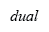Relational Algebra Tree: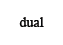Output:

```DUMMY
----------
X
```

The following command displays the number of rows of DUAL table :

``````SELECT COUNT(*) FROM DUAL;
``````

Relational Algebra Expression: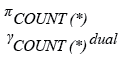Relational Algebra Tree: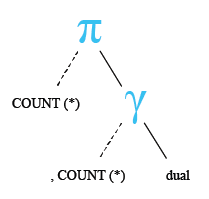Output:

```  COUNT(*)
----------
1
```

The following command displays the string value from the DUAL table :

``````SELECT 'ABCDEF12345' FROM DUAL;
``````

Relational Algebra Expression: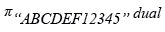Relational Algebra Tree: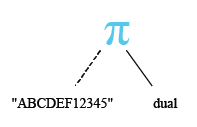Output:

```'ABCDEF1234
-----------
ABCDEF12345
```

The following command displays the numeric value from the DUAL table :

``````SELECT 123792.52 FROM DUAL;
``````

Relational Algebra Expression: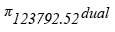Relational Algebra Tree: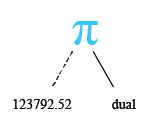Output:

``` 123792.52
----------
123792.52
```

The following command tries to delete all rows from the DUAL table :

``````DELETE FROM DUAL;
``````

Output:

```DELETE FROM DUAL
*
ERROR at line 1:
ORA-01031: insufficient privileges
```

The following command tries to remove all rows from the DUAL table :

``````TRUNCATE TABLE DUAL;
``````

Note : The DELETE command is used to remove rows from a table. After performing a DELETE operation you need to COMMIT or ROLLBACK the transaction to make the change permanent or to undo it. TRUNCATE removes all rows from a table. The operation cannot be rolled back.

Output:

```TRUNCATE TABLE DUAL
*
ERROR at line 1:
ORA-00942: table or view does not exist
```

The following command select two rows from dual :

``````SELECT dummy FROM DUAL
UNION ALL
SELECT dummy FROM DUAL;
``````

Relational Algebra Expression: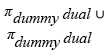Relational Algebra Tree: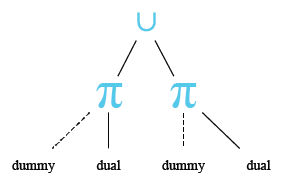Output

```DUMMY
----------
X
X
```

Example - 1

You can also check the system date from the DUAL table using the following statement :

``````SELECT sysdate FROM DUAL ;
``````

Relational Algebra Expression: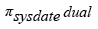Relational Algebra Tree: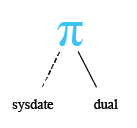Output:

```SYSDATE
---------
11-DEC-10
```

Example - 2

You can also check the arithmetic calculation from the DUAL table using the following statement :

``````SELECT 15+10-5*5/5 FROM DUAL;
``````

Relational Algebra Expression: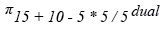Relational Algebra Tree: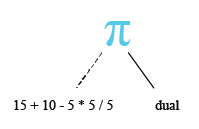Output:

```15+10-5*5/5
-----------
20
```

Example - 3

Following code display the numbers 1..10 from DUAL :

``````SELECT level
FROM DUAL
CONNECT BY level <=10;
``````

Output:

```     LEVEL
----------
1
2
3
4
5
6
7
8
9
10
```

Example - 4

In the following code, DUAL involves the use of decode with NULL.

``````SELECT decode(null,null,1,0)
FROM DUAL;
``````

Output:

```DECODE(NULL,NULL,1,0)
---------------------
1
```

## DUAL table : Oracle vs MySQL

We have already learned that DUAL is a special one row one column table. For Oracle, it is useful because Oracle doesn't allow statements like :

``````SELECT 15+10-5*5/5;
``````

Relational Algebra Expression:Relational Algebra Tree:Output:

```SELECT 15+10-5*5/5
*
ERROR at line 1:
```

But the following command will execute (see the output of the previous example) :

``````SELECT 15+10-5*5/5 FROM DUAL;
``````

In case of MySQL the following command will execute :

``````SELECT 15+10-5*5/5;
``````

Output: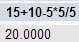The following table shows the uses of dummy table in standard DBMS.

DBMS Dummy-table concept
MSSQL No dummy-table concept.
MySQL No dummy-table concept.
Oracle Dummy-table : DUAL.
Informix Since version 11.10, a dummy table has been included : sysmaster:sysdual
PostgreSQL No dummy-table concept.
DB2 Dummy-table : SYSIBM.SYSDUMMY1

Practice SQL Exercises

﻿

## SQL: Tips of the Day

How to check if a table exists in a given schema?

```SELECT EXISTS (
SELECT FROM information_schema.tables
WHERE  table_schema = 'schema_name'
AND    table_name   = 'table_name'
);
```

Ref: https://bit.ly/3MjkoYD